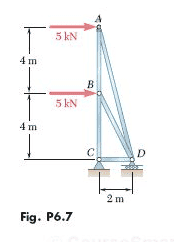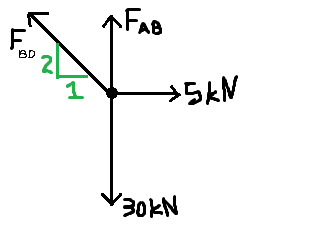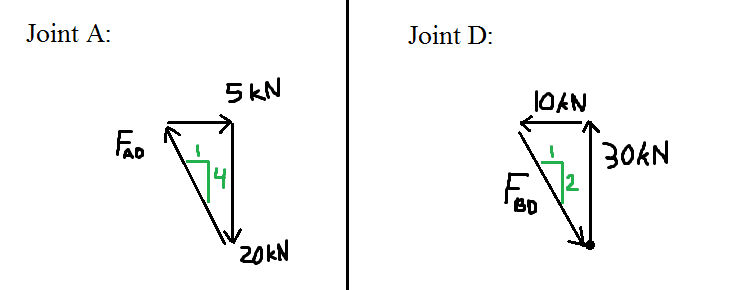# Statics: Forces in a truss, method of joints

## Homework Statement

Using the method of joints, determine the force in each member of the truss shown. State whether each member is in tension or compression.ƩFx=0
ƩFy=0
ƩM=0

## The Attempt at a Solution

I got the forces at C and D by using the equilibrium equations for the entire truss:
D = 30 kN ↑
Cx = 10 kN ←
Cy = 30 kN ↓

By anlaysis of the forces at joint C, I obtained:
FCD = 10 kN T
FBC = 30 kN T

My problem is joint with joint B. Here is my diagram of its forces:ƩFx=0: 5 - (1/√5)*FBD = 0
FBD = 11.18 kN C

ƩFy=0: FAB - 30 + (2/√5)*11.18 = 0
FAB = 20 kN T

I got FAB correct, but FBD is supposed to be 30 kN T

## Answers and Replies

Since FAB was correct, and your answer to FAB depended on your "incorrect" answer for FBD, I find it hard to believe that FAB was right and FBD was wrong.

Your reasoning looks sound and your understanding seems strong. At this point, I would start to be suspicious of whether the answer key you are using is correct. In my sophomore level Differential Equations class, it took us about a month to realize that the "solutions manual" kept giving incorrect solutions and that we were actually doing the problems correctly.

Since FAB was correct, and your answer to FAB depended on your "incorrect" answer for FBD, I find it hard to believe that FAB was right and FBD was wrong.

Your reasoning looks sound and your understanding seems strong. At this point, I would start to be suspicious of whether the answer key you are using is correct. In my sophomore level Differential Equations class, it took us about a month to realize that the "solutions manual" kept giving incorrect solutions and that we were actually doing the problems correctly.

I thought this was the case, but then I went ahead and found FAD using joint A, and took a look at joint D to see if I got the same answer for FBD.

Diagrams:Joint A:
5 = FAD/√5
FAD = 20.6 kN
This is correct according to the book.

Joint D:
This one is strange because the ratios don't add up
30/2 ≠ 10/1
At least one of the forces must be wrong, I can't figure it out.

EDIT: I realize I forgot to include FAD on joint D, going to try that now.

EDIT 2: It seems you're right. I solved joint D with all the forces and got my original answer. Must be a typo, my other answers were "correct" and I haven't had any other inconsistencies with other problems. In any case I'll keep this in mind.

Last edited:
Force AD Acting on Joint D?

You seem to have the force from the ground on D, the force from C on D and the force from B on D in your diagram. What about the force from A?